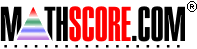Math Practice Online > free > lessons > Florida > 6th grade > Single Variable Equations 2

## Single Variable Equations 2

Solve single variable equations in one or two steps. You may need pencil and paper.

 Sample Problems for Single Variable Equations 2 Lesson for Single Variable Equations 2

### This topic aligns to the following state standards

Grade 7: Alg 4. Uses pictures, models, manipulatives or other strategies to solve one-step and simple multi- step linear equations.
Grade 8: Alg 3. Solves single- and multiple-step linear equations and inequalities in concrete or abstract form.

Copyright Accurate Learning Systems Corporation 2008.